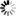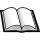Dr. S. R. Lasker Library Online Catalogue

Normal view

# Applied numerical methods with MATLAB for engineers and scientists / Steven C. Chapra.

Material type:TextLanguage: English Publication details: c2008. Edition: 2nd edDescription: xx, 588 p. : ill. ; 25 cmISBN: 007313290X (acidfree paper); 9780071259217 ; 007125921XDDC classification: 518 LOC classification: QA297 | .C4185 2008Online resources: WorldCat details | Ebook Fulltext
Contents:
TOC Mathematical modeling, numberical methods, and problem solving -- MATLAB fundamentals -- Programming with MATLAB -- Roundoff and truncation errors -- Roots: bracketing methods -- Roots: open methods -- Optimization -- Linear algebraic equations and matrics -- Gauss elimination -- LU factorization -- Matrix inverse and condition -- Iterative methods -- Linear regression -- General linear least-squares and nonlinear regression -- Polynomial interpolation -- Splines and piecewise interpolation -- Numerical integration formulas -- Numerical integration of functions -- Numerical differentiation -- Initial-value problems -- Adaptive methods and stiff systems -- Boundary-value problems
Summary: Summary: Applied Numerical Methods with MATLAB for Engineering and Science is the newest book by best-selling author Steve Chapra. The new text uses MATLAB as the primary computing environment and focuses on applications. Theory is included only when it has direct use to the student; i.e., when theory informs the concepts. Information relating to the limitations of methods and to choosing among different methods is stressed Read more...
Tags from this library: No tags from this library for this title.
Star ratingsAverage rating: 0.0 (0 votes)
Holdings
Item type Current library Collection Call number Copy number Status Date due Barcode Item holdsE-Book
E-book
Non-fiction 518 CHA 2008 (Browse shelf(Opens below)) Not for loanText
Reserve Section
Non-fiction 518 CHA 2008 (Browse shelf(Opens below)) C-1 Not For Loan 20679Text
Circulation Section
Non-fiction 518 CHA 2008 (Browse shelf(Opens below)) C-2 Available 20680
Total holds: 0

Online version:
Chapra, Steven C.
Applied numerical methods with MATLAB for engineers and scientists.
Boston : McGraw-Hill Higher Education, c2008
(OCoLC)767688956

Includes bibliographical references and index.

TOC Mathematical modeling, numberical methods, and problem solving --
MATLAB fundamentals --
Programming with MATLAB --
Roundoff and truncation errors --
Roots: bracketing methods --
Roots: open methods --
Optimization --
Linear algebraic equations and matrics --
Gauss elimination --
LU factorization --
Matrix inverse and condition --
Iterative methods --
Linear regression --
General linear least-squares and nonlinear regression --
Polynomial interpolation --
Splines and piecewise interpolation --
Numerical integration formulas --
Numerical integration of functions --
Numerical differentiation --
Initial-value problems --
Adaptive methods and stiff systems --
Boundary-value problems

Summary:
Applied Numerical Methods with MATLAB for Engineering and Science is the newest book by best-selling author Steve Chapra. The new text uses MATLAB as the primary computing environment and focuses on applications. Theory is included only when it has direct use to the student; i.e., when theory informs the concepts. Information relating to the limitations of methods and to choosing among different methods is stressed Read more...

APE

Saifun Momota

There are no comments on this title.